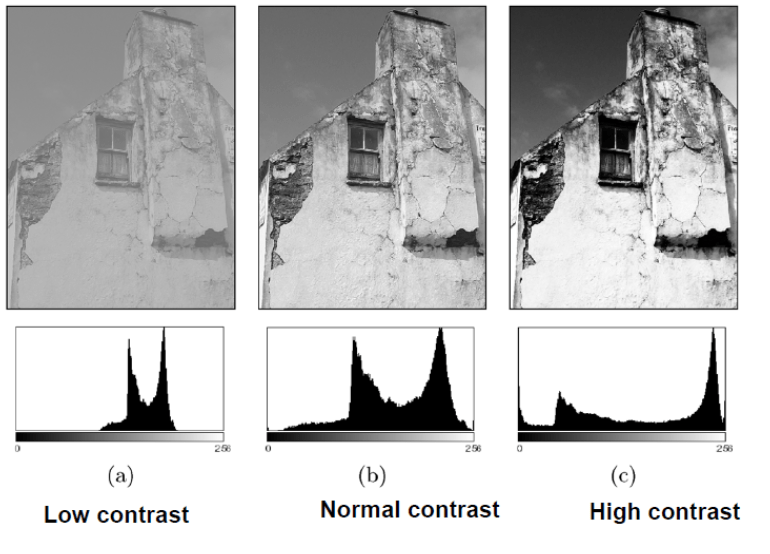# Basic Relationships Between Pixels

### Basic Relationships Between Pixels

• Neighborhood
• Paths
• Connectivity
• Regions
• Boundaries

#### Neighbors of a pixel – N4(p)

• Any pixel p(x, y) has two vertical and two horizontal neighbors, given by

(x+1, y),

(x-1, y),

(x, y+1),

(x, y-1)

• This set of pixels are called the 4-neighbors of P, and is denoted by N4(P).
 x , y+1 x-1 , y x,y x+1 , y x , y-1

#### Neighbors of a pixel – ND(p)

• Any pixel p(x, y) has four diagonal neighbors, given by

(x+1, y+1), (x+1, y-1), (x-1, y+1), (x-1 ,y-1)

• This set is denoted by ND(p).
 x-1 , y+1 x+1, y+1 x,y x-1, y-1 x+1,y-1

#### Neighbors of a pixel – N8(p)

• ND(p) and N4(p) are together known as 8-Neighbors and are denoted by N8(p)
• ND(p) U N4(p) = N8(p)
• What about when p(x,y) is a border pixel of the image ?
 x-1,y+1 x,y+1 x+1,y+1 x-1,y x,y x+1,y x-1,y-1 x,y-1 x+1, y-1

• Let V be the set of intensity values used to define adjacency
• For binary images à V = {1}
• A particular grayscale image à V = {1,3,5,…,251,253,255}
• 4-adjacency: Two pixels p and q with values from V are 4-adjacent if q is in the set N4(p).
• 8-adjacency: Two pixels p and q with values from V are 8-adjacent if q is in the set N8(p).
• m-adjacency: Two pixels p and q with values from V are m-adjacent if,

q is in N4(p)

OR

q is in ND(p) AND N4(p)N4(q) has no pixels whose values are from V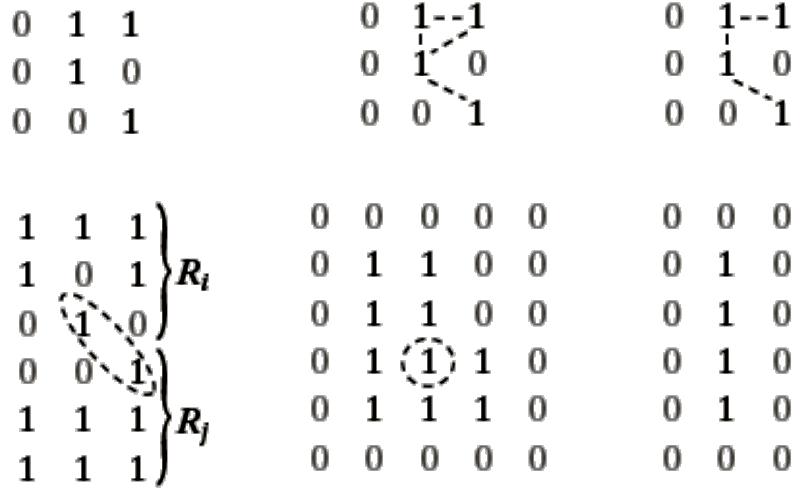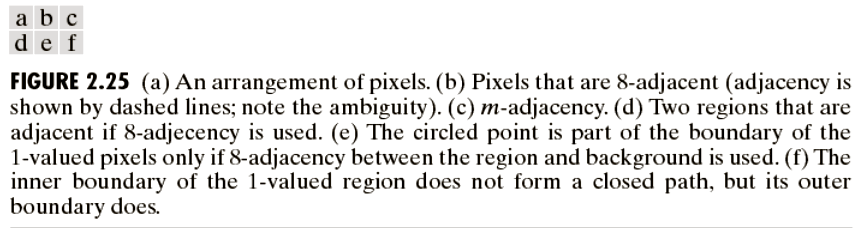#### Path

• set of pixels lying in some adjacency definition
• path length ?
• Number of pixels involved

#### Connectivity

• Let Sà subset of pixels in an image
• Two pixels p and q are said to be connected in S if there exist a path between them consisting entirely of pixels in S.
• For any pixel p in S the set of pixels that are connected to it in S is called connected component of S.
• If S has only one connected component, then it is called connected set.

#### Region

• A connected set is also called a Region.
• Two regions (let Ri and Rj) are said to be adjacent if their union forms a connected set. Adjacent Regions or joint regions
• Regions that are not adjacent are said to be disjoint regions.
• 4- and 8-adjacency is considered when referring to regions (author)
• Discussing a particular region, type of adjacency must be specified.
• Fig2.25d the two regions are adjacent only if 8-adjacency is considered

#### Foreground and Background

• Suppose an image contain K disjoint regions Rk , k=1,2,3,…K, none of which touches the image border
• Let Ru denote the union of all the K regions.
• Let (Ru)c denote its compliment.
• We call all the points in Ru the foreground and all the points in (Ru)c the background

#### Boundary

• The boundary (border or contour) of a region R is the set of points that are adjacent to the points in the complement of R.
• Set of pixels in the region that have at least one background neighbor.
• The boundary of the region R is the set of pixels in the region that have one or more neighbors that are not in R.
• Inner Border: Border of Foreground
• Outer Border: Border of Background
• If R happens to be entire Image?
• There is a difference between boundary and edge in Digital Image Paradigm. The author refers this discussion to chapter 10.

#### Distance Measures

• Euclidean Distance: De(p, q) = [(x-s)2 + (y-t)2]1/2
•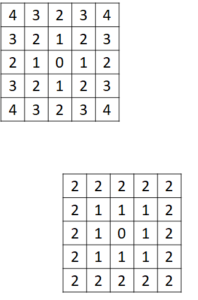• City Block Distance: D4(p, q) = |x-s| + |y-t|
• Chess Board Distance: D8(p, q) = max(|x-s|, |y-t|)

#### Sample Problem from exercise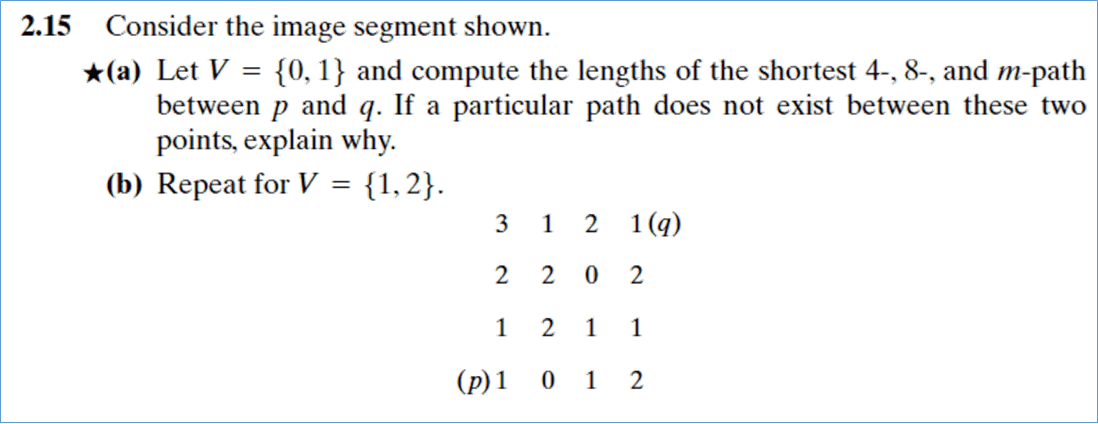#### Histogram Representation

• Histograms plots how many times (frequency) each intensity value in image occurs
• Image below (left) has 256 distinct gray levels (8 bits)
• Histogram (right) shows frequency (how many times) each gray level occurs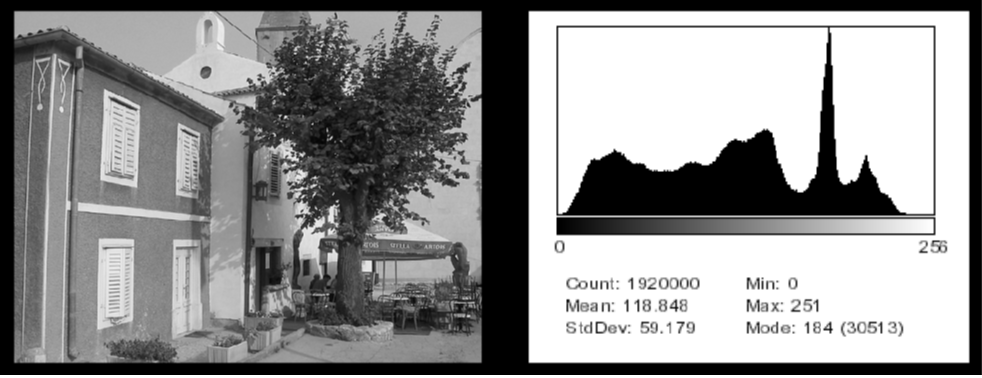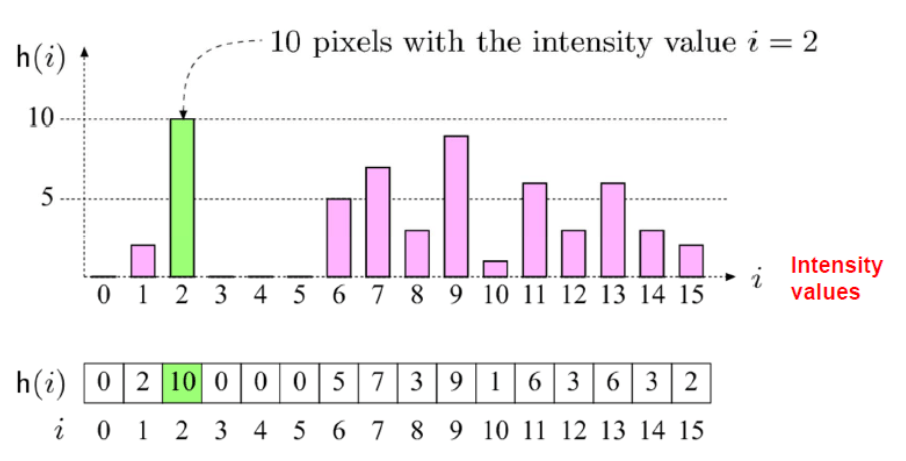• E.g. K = 16, 10 pixels have intensity value = 2
• Only statistical information
• No indication of location of pixels

#### Rough guess about the histogram of these images ?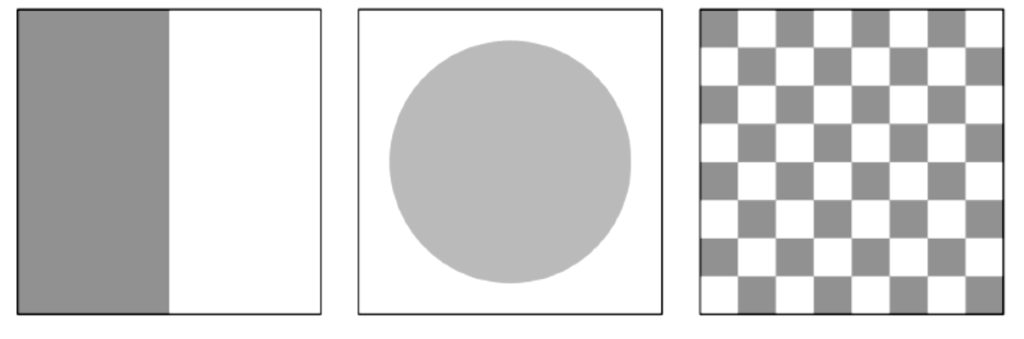#### Histogram Representation

• Different images can have same histogram
• 3 images below have same histogram
• Half of pixels are gray, half are white
• Same histogram = Same statistics
• Distribution of intensities could be different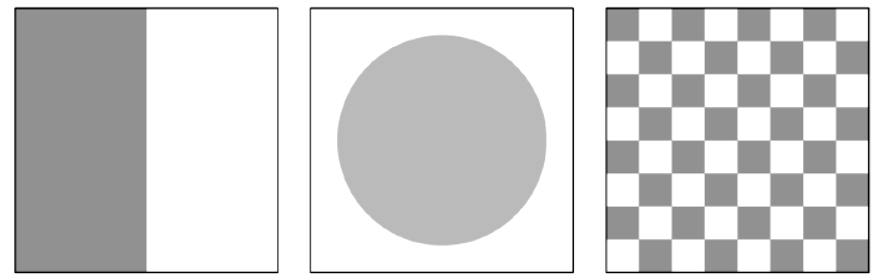#### Histogram Representation

• Many cameras display real time histograms of scene
• Helps taking pictures according to your requirement
• Also easier to detect types of processing applied to image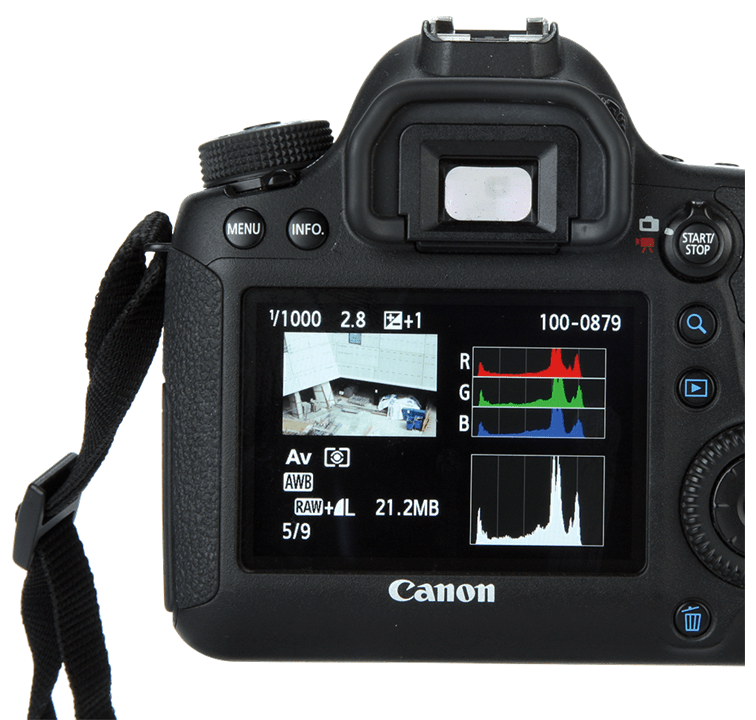?

• Can we reconstruct image from histogram ?

#### Histogram

• Histograms help detect image acquisition issues
• Histogram representation of an image can be useful in following characteristics of an image.
• Exposure: amount of light per unit area reaching the image sensor
• Brightness: average intensity of all pixels in image
• Contrast: difference of foreground and background (objects distinction)
• Dynamic Range: Number of distinct pixels in image
• Artifacts: Image alteration after it is being captured

#### Histogram Representation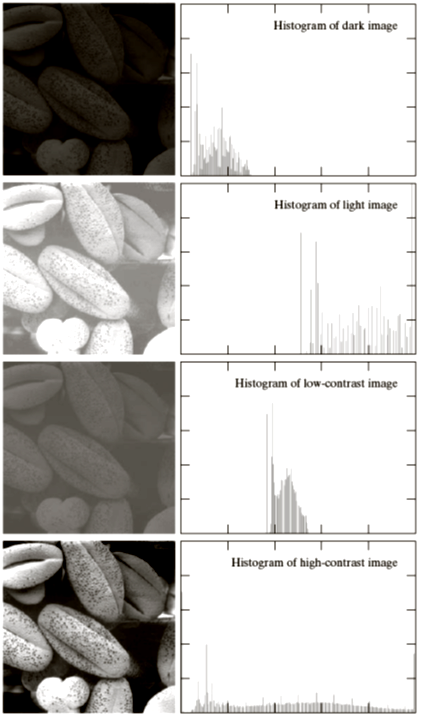Four basic image types: dark, light, low contrast, high contrast and their corresponding histograms.

#### Histogram Representation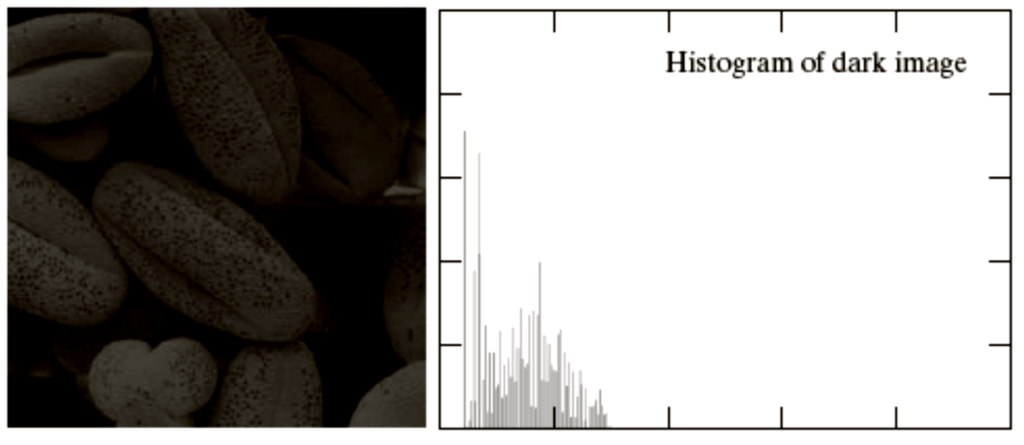#### Histogram Representation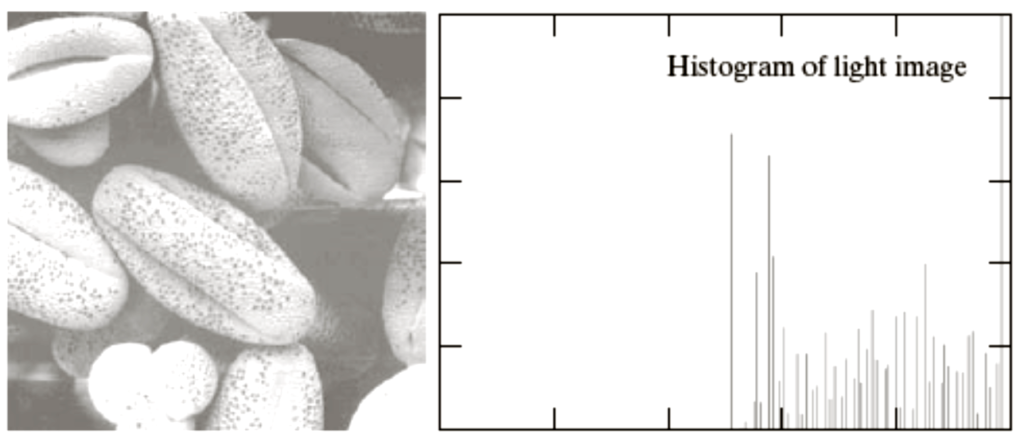#### Histogram Representation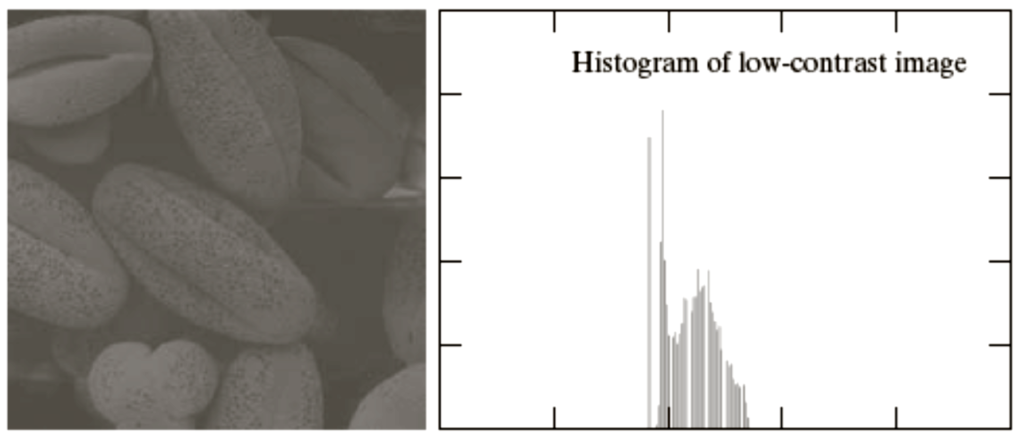#### Histogram Representation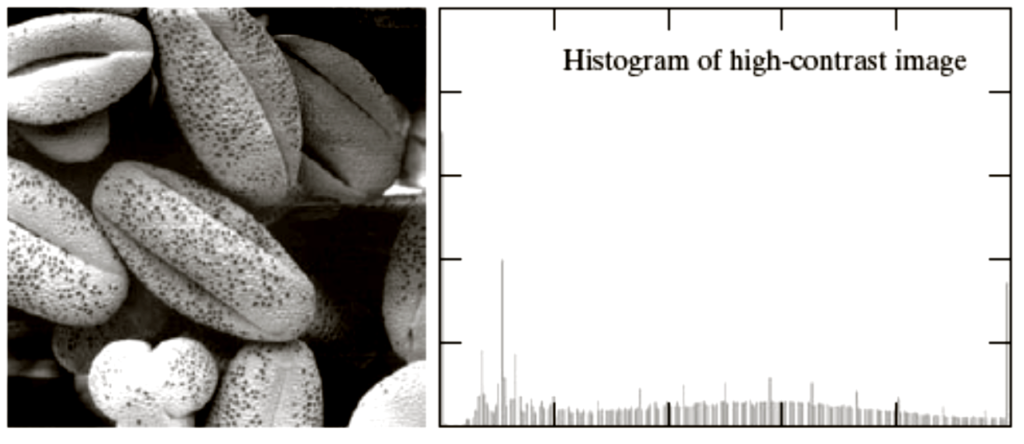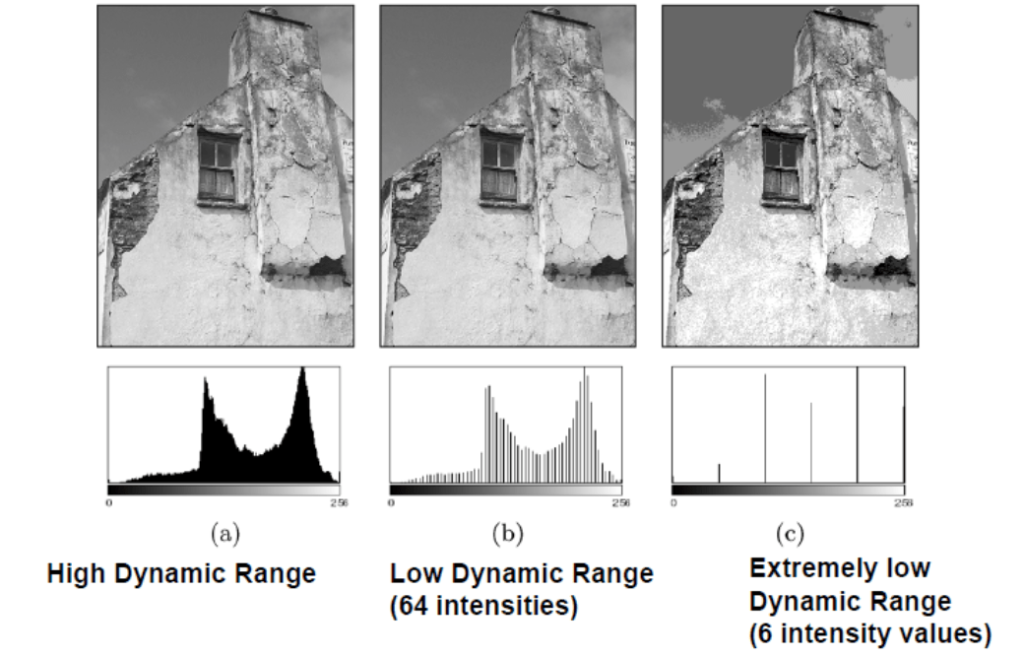#### Contrast

• Good Contrast?